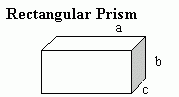Email us to get an instant 20% discount on highly effective K-12 Math & English kwizNET Programs!

#### Online Quiz (WorksheetABCD)

Questions Per Quiz = 2 4 6 8 10

### Grade 5 - Mathematics10.1 Rectangular Prisms and CubesA square prism or a cube is a three-dimensional solid object bounded by six square faces or sides, with three meeting at each vertex. It is a special kind of square prism.A rectangular prism is a three-dimensional solid object which has six faces that are rectangles. It is a prism because it has the same cross-section along a length. Example: What is the name of this shape?Answer: Rectangular Prism Directions: Answer the following questions. Also find at least 10 examples of cubes and rectangular prisms found around you.
 Q 1: A rectangular Prism has ___ faces that are rectangles.765 Q 2: What is the name of the shape shown.Rectangular PrismCubeSquare Prism Q 3: What is a special kind of rectangular prism with all its edges having same length and all its faces congruent.solidcubesphere Q 4: What is the name of the shape shown.Rectangular PrismCubeTriangular Prism Question 5: This question is available to subscribers only! Question 6: This question is available to subscribers only!